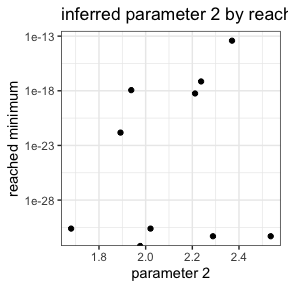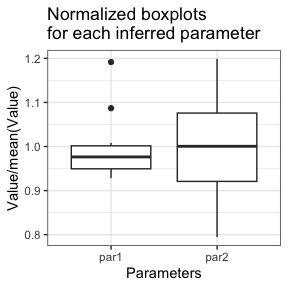# Examples - flimo

## Overview

Flimo (Fixed Landscape Inference MethOd) is a likelihood-free inference method for stochastic models. It is based on simple simulations of the process under study with a specific management of the randomness. This makes it possible to use deterministic optimization algorithms to find the optimal parameters in the sense of summary statistics.

This document presents two small examples to illustrate how the method works. You can find more details on the git page of the project: https://metabarcoding.org/flimo.

Inference is possible in R but is more efficient in the Julia language for non-trivial models. This Julia mode uses the ‘Jflimo’ package available on the git page of the project: https://metabarcoding.org/flimo.

## Example 1 : Poisson Distribution

Five Poisson variables with parameter $$\theta = 100$$ are drawn. We try to find this value by comparing mean of 10 simulated Poisson variables with the observed data. The distance between the summary statistics is :

$dsumstats(\theta) =\left( \widehat{\mathbb{E}[X|\theta]}-\overline{X^{data}}\right)^2=\left(\frac{1}{n_{sim}}\sum_{i=1}^{n_{sim}}X_i^\theta - \frac{1}{n_{data}}\sum_{i=1}^{n_{data}}X_i^{data}\right)^2$

With the Normal approximation, Poisson distribution is replaced by a Normal distribution with same mean and variance :

$\mathcal{P}(\theta) \leftarrow \mathcal{N}(\mu = \theta, \sigma^2 = \theta)$

#Setup
set.seed(1)

#Create data

Theta_true1 <- 100 #data parameter
n1 <- 5 #data size

simulator1 <- function(Theta, n){
#classical random simulator
rpois(n, lambda = Theta)
}

data1 <- simulator1(Theta_true1, n1)

#Simulations with quantiles
#See README to know how to build this simulator

ndraw1 <- n1 #number of random draws for one simulation

check_simulator(simulator1, ndraw1, 0, 200)
#>  FALSE

simulatorQ1 <- function(Theta, quantiles){
qpois(quantiles, lambda = Theta)
}
check_simulator(simulatorQ1, ndraw1, 0, 200)
#>  TRUE

#With Normal approximation
simulatorQ1N <- function(Theta, quantiles){
qnorm(quantiles, mean = Theta, sd = sqrt(Theta))
}

check_simulator(simulatorQ1N, ndraw1, 0, 200)
#>  TRUE

#Quantile-simulator with Normal approximation

#First simulations

Theta11 <- 50
Theta21 <- 200

nsim1 <- 10

quantiles1 <- matrix(runif(ndraw1*nsim1), nrow = nsim1)

#Just one simulation
simulatorQ1(Theta11, quantiles1[1,])
#>  49 43 52 45 49
#No random effect:
simulatorQ1(Theta11, quantiles1[1,])
#>  49 43 52 45 49

#Independent values:
simulatorQ1(Theta11, quantiles1[2,])
#>  43 55 61 54 50

#Matrix of nsim simulations
simu11 <- simulatorQ1(Theta11, quantiles1)
simu21 <- simulatorQ1(Theta21, quantiles1)

#Sample Comparison: summary statistics

dsumstats1 <- function(simulations, data){
#simulations : 2D array
#data : 1D array
mean_simu <- mean(rowMeans(simulations))
mean_data <- mean(data)
(mean_simu-mean_data)^2
}

Plot the objective function:

#Objective by parameter:
plot_objective(ndraw1, data1, dsumstats1, simulatorQ1,
nsim = nsim1, lower = 0, upper = 200)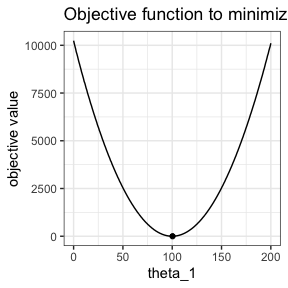#Objective with Normal approximation :
plot_objective(ndraw1, data1, dsumstats1, simulatorQ1N,
nsim = nsim1, lower = 0, upper = 200)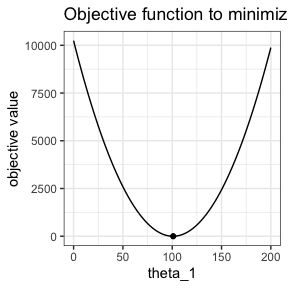#both plots
#We use same quantiles for both

quantiles1 <- matrix(runif(ndraw1*nsim1), nrow = nsim1)

p <- plot_objective(data = data1,
dsumstats = dsumstats1,
simulatorQ = simulatorQ1,
lower = 0, upper = 200, quantiles = quantiles1)

plot_objective(data = data1,
dsumstats = dsumstats1,
simulatorQ = simulatorQ1N,
lower = 0, upper = 200,
visualize_min = FALSE,
add_to_plot = p, quantiles = quantiles1)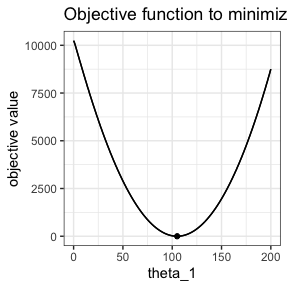Locally, Poisson quantiles and then objective function are constant by pieces. Let’s compare it with normal approximation.

p <- plot_objective(data = data1,
dsumstats = dsumstats1,
simulatorQ = simulatorQ1,
lower = 71, upper = 72,
visualize_min = FALSE, quantiles = quantiles1)

plot_objective(ndraw1, data1, dsumstats1, simulatorQ1N,
lower = 71, upper = 72,
nsim = nsim1,
visualize_min = FALSE, add_to_plot = p,
quantiles = quantiles1)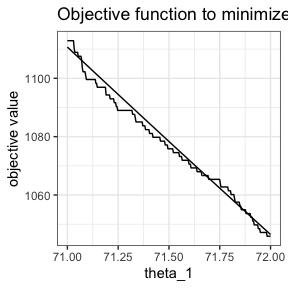### Use of flimo

There are optimization issues in R for normalized process and Theta close to 0. Lower bound set to 1.

#Optimization with normal approximation of Poisson distribution

#Default mode: full R

system.time(optim1R <- flimoptim(ndraw1, data1, dsumstats1, simulatorQ1N,
ninfer = 10,
nsim = nsim1,
lower = 1, upper = 1000,
randomTheta0 = TRUE))
#>    user  system elapsed
#>   0.014   0.000   0.014
optim1R
#> optimization Result
#> Mode : R
#> method : L-BFGS-B
#> Number of inferences : 10
#> Convergence : 10/10
#> Mean of minimizer :
#> 100.659429936366
#> Best minimizer :
#> 101.125271999801
#> Reached minima : from 8.27180612553028e-25 to 6.61968703244348e-20
#> Median time by inference 0.00103199481964111
#> optimization Result
#> Mode : R
#> method : L-BFGS-B
#> Number of inferences : 10
#> Convergence : 10/10
#> Mean of minimizer :
#> 100.659429936366
#> Best minimizer :
#> 101.125271999801
#> Reached minima : from 8.27180612553028e-25 to 6.61968703244348e-20
#> Median time by inference 0.00103199481964111
summary(optim1R)
#> $Mode #>  "R" #> #>$method
#>  "L-BFGS-B"
#>
#> $number_inferences #>  10 #> #>$number_converged
#>  10
#>
#> $minimizer #> par1 #> Min. : 99.29 #> 1st Qu.: 99.76 #> Median :100.72 #> Mean :100.66 #> 3rd Qu.:101.09 #> Max. :102.62 #> #>$minimum
#>      Min.   1st Qu.    Median      Mean   3rd Qu.      Max.
#> 8.300e-25 5.614e-23 3.429e-22 6.947e-21 5.661e-22 6.620e-20
#>
#> $median_time_inference #>  0.001031995 attributes(optim1R) #>$names
#>   "mode"      "method"    "AD"        "minimizer" "minimum"   "converged"
#>   "initial_x" "f_calls"   "g_calls"   "message"   "time_run"
#>
#> $class #>  "flimo_result" plot(optim1R)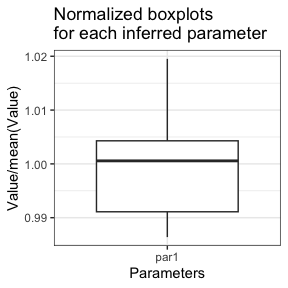#Version with objective function provided obj1 <- function(Theta, quantiles, data = data1){ simulations <- simulatorQ1N(Theta, quantiles) dsumstats1(simulations, data) } system.time(optim1Rbis <- flimoptim(ndraw1, obj = obj1, ninfer = 10, nsim = nsim1, lower = 1, upper = 1000, randomTheta0 = TRUE)) #> user system elapsed #> 0.007 0.000 0.007 To use the Julia mode, see Readme on the git page of the project. ## Example 2 : Normal Distribution Five normal variables with mean = 0 and sd = 1 are drawn. We try to find these mean/sd values by comparing mean and sd of 10 simulated normal variables with the observed data. The summary statistic is : $dsumstats(\theta) =\left( \widehat{\mathbb{E}[X|\theta]}-\overline{X^{data}}\right)^2 + \left( \widehat{\sigma(X|\theta)}-\sigma(X^{data})\right)^2$ #Setup set.seed(1) #Create data Theta_true2 <- c(3, 2) #data parameter n2 <- 5 #data size simulator2 <- function(Theta, n){ #classical random simulator rnorm(n, mean = Theta, sd = Theta) } data2 <- simulator2(Theta_true2, n2) #Simulations with quantiles #See README to know how to build this simulator simulatorQ2 <- function(Theta, quantiles){ qnorm(quantiles, mean = Theta, sd = Theta) } ndraw2 <- 5 check_simulator(simulatorQ2, ndraw2, c(0, 0), c(10, 10)) #>  TRUE check_simulator(simulator2, ndraw2, c(0, 0), c(10, 10)) #>  FALSE dsumstats2 <-function(simulations, data){ mean_simu <- mean(rowMeans(simulations)) mean_data <- mean(data) sd_simu <-mean(apply(simulations, 1, sd)) sd_data <- sd(data) (mean_simu-mean_data)^2+(sd_simu-sd_data)^2 } nsim2 <- 10 plot_objective(ndraw2, data2, dsumstats2, simulatorQ2, index = 1, other_param = c(1, 2, 10), nsim = nsim2, lower = -5, upper = 10)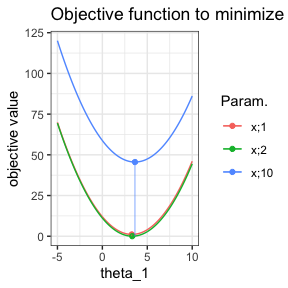#Optimization #Default mode: full R optim2R <- flimoptim(ndraw2, data2, dsumstats2, simulatorQ2, ninfer = 10, nsim = nsim2, lower = c(-5, 0), upper = c(10, 10), randomTheta0 = TRUE) optim2R #> optimization Result #> Mode : R #> method : L-BFGS-B #> Number of inferences : 10 #> Convergence : 10/10 #> Mean of minimizer : #> 3.20567648680977 2.11520187608635 #> Best minimizer : #> 3.14574674163785 1.97643436033205 #> Reached minima : from 0 to 3.8290401805314e-14 #> Median time by inference 0.006988525390625 #> optimization Result #> Mode : R #> method : L-BFGS-B #> Number of inferences : 10 #> Convergence : 10/10 #> Mean of minimizer : #> 3.20567648680977 2.11520187608635 #> Best minimizer : #> 3.14574674163785 1.97643436033205 #> Reached minima : from 0 to 3.8290401805314e-14 #> Median time by inference 0.006988525390625 summary(optim2R) #>$Mode
#>  "R"
#>
#> $method #>  "L-BFGS-B" #> #>$number_inferences
#>  10
#>
#> $number_converged #>  10 #> #>$minimizer
#>       par1            par2
#>  Min.   :2.975   Min.   :1.681
#>  1st Qu.:3.044   1st Qu.:1.948
#>  Median :3.130   Median :2.116
#>  Mean   :3.206   Mean   :2.115
#>  3rd Qu.:3.211   3rd Qu.:2.276
#>  Max.   :3.820   Max.   :2.536
#>
#> $minimum #> Min. 1st Qu. Median Mean 3rd Qu. Max. #> 0.000e+00 0.000e+00 0.000e+00 3.830e-15 1.000e-18 3.829e-14 #> #>$median_time_inference
#>  0.006988525
plot(optim2R, pairwise_par = TRUE, hist = TRUE, par_minimum = TRUE)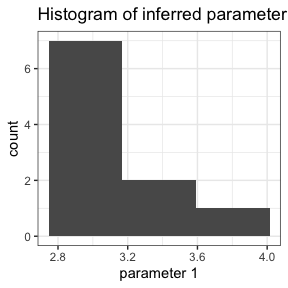#> Warning: Transformation introduced infinite values in continuous y-axis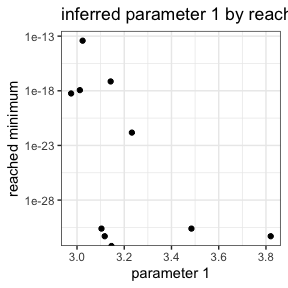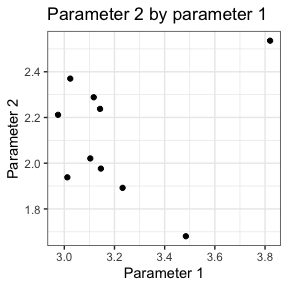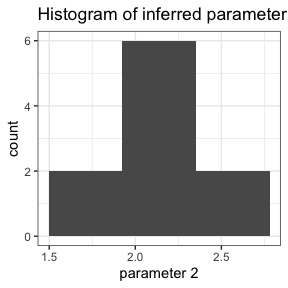#> Warning: Transformation introduced infinite values in continuous y-axis